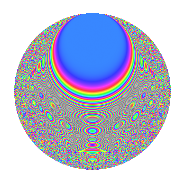Properties

 Label 6011.2.a.eLevel 6011 Weight 2 Character orbit 6011.a Self dual Yes Analytic conductor 47.998 Analytic rank 1 Dimension 221 CM No

Related objects

Newspace parameters

 Level: $$N$$ = $$6011$$ Weight: $$k$$ = $$2$$ Character orbit: $$[\chi]$$ = 6011.a (trivial)

Newform invariants

 Self dual: Yes Analytic conductor: $$47.998076655$$ Analytic rank: $$1$$ Dimension: $$221$$ Fricke sign: $$1$$ Sato-Tate group: $\mathrm{SU}(2)$

$q$-expansion

The dimension is sufficiently large that we do not compute an algebraic $$q$$-expansion, but we have computed the trace expansion.

 $$\operatorname{Tr}(f)(q) =$$ $$221q$$ $$\mathstrut -\mathstrut 15q^{2}$$ $$\mathstrut -\mathstrut 17q^{3}$$ $$\mathstrut +\mathstrut 189q^{4}$$ $$\mathstrut -\mathstrut 32q^{5}$$ $$\mathstrut -\mathstrut 33q^{6}$$ $$\mathstrut -\mathstrut 40q^{7}$$ $$\mathstrut -\mathstrut 39q^{8}$$ $$\mathstrut +\mathstrut 176q^{9}$$ $$\mathstrut +\mathstrut O(q^{10})$$ $$\operatorname{Tr}(f)(q) =$$ $$221q$$ $$\mathstrut -\mathstrut 15q^{2}$$ $$\mathstrut -\mathstrut 17q^{3}$$ $$\mathstrut +\mathstrut 189q^{4}$$ $$\mathstrut -\mathstrut 32q^{5}$$ $$\mathstrut -\mathstrut 33q^{6}$$ $$\mathstrut -\mathstrut 40q^{7}$$ $$\mathstrut -\mathstrut 39q^{8}$$ $$\mathstrut +\mathstrut 176q^{9}$$ $$\mathstrut -\mathstrut 61q^{10}$$ $$\mathstrut -\mathstrut 50q^{11}$$ $$\mathstrut -\mathstrut 43q^{12}$$ $$\mathstrut -\mathstrut 87q^{13}$$ $$\mathstrut -\mathstrut 41q^{14}$$ $$\mathstrut -\mathstrut 62q^{15}$$ $$\mathstrut +\mathstrut 129q^{16}$$ $$\mathstrut -\mathstrut 29q^{17}$$ $$\mathstrut -\mathstrut 61q^{18}$$ $$\mathstrut -\mathstrut 107q^{19}$$ $$\mathstrut -\mathstrut 59q^{20}$$ $$\mathstrut -\mathstrut 163q^{21}$$ $$\mathstrut -\mathstrut 70q^{22}$$ $$\mathstrut -\mathstrut 31q^{23}$$ $$\mathstrut -\mathstrut 98q^{24}$$ $$\mathstrut +\mathstrut 119q^{25}$$ $$\mathstrut -\mathstrut 23q^{26}$$ $$\mathstrut -\mathstrut 41q^{27}$$ $$\mathstrut -\mathstrut 112q^{28}$$ $$\mathstrut -\mathstrut 152q^{29}$$ $$\mathstrut -\mathstrut 66q^{30}$$ $$\mathstrut -\mathstrut 117q^{31}$$ $$\mathstrut -\mathstrut 93q^{32}$$ $$\mathstrut -\mathstrut 60q^{33}$$ $$\mathstrut -\mathstrut 80q^{34}$$ $$\mathstrut -\mathstrut 21q^{35}$$ $$\mathstrut +\mathstrut 92q^{36}$$ $$\mathstrut -\mathstrut 231q^{37}$$ $$\mathstrut +\mathstrut 2q^{38}$$ $$\mathstrut -\mathstrut 81q^{39}$$ $$\mathstrut -\mathstrut 143q^{40}$$ $$\mathstrut -\mathstrut 81q^{41}$$ $$\mathstrut -\mathstrut 6q^{42}$$ $$\mathstrut -\mathstrut 126q^{43}$$ $$\mathstrut -\mathstrut 115q^{44}$$ $$\mathstrut -\mathstrut 156q^{45}$$ $$\mathstrut -\mathstrut 205q^{46}$$ $$\mathstrut -\mathstrut 4q^{47}$$ $$\mathstrut -\mathstrut 55q^{48}$$ $$\mathstrut +\mathstrut 103q^{49}$$ $$\mathstrut -\mathstrut 61q^{50}$$ $$\mathstrut -\mathstrut 106q^{51}$$ $$\mathstrut -\mathstrut 164q^{52}$$ $$\mathstrut -\mathstrut 87q^{53}$$ $$\mathstrut -\mathstrut 110q^{54}$$ $$\mathstrut -\mathstrut 62q^{55}$$ $$\mathstrut -\mathstrut 73q^{56}$$ $$\mathstrut -\mathstrut 136q^{57}$$ $$\mathstrut -\mathstrut 128q^{58}$$ $$\mathstrut -\mathstrut 76q^{59}$$ $$\mathstrut -\mathstrut 148q^{60}$$ $$\mathstrut -\mathstrut 345q^{61}$$ $$\mathstrut +\mathstrut 5q^{62}$$ $$\mathstrut -\mathstrut 74q^{63}$$ $$\mathstrut -\mathstrut 25q^{64}$$ $$\mathstrut -\mathstrut 110q^{65}$$ $$\mathstrut -\mathstrut 34q^{66}$$ $$\mathstrut -\mathstrut 104q^{67}$$ $$\mathstrut -\mathstrut 48q^{68}$$ $$\mathstrut -\mathstrut 133q^{69}$$ $$\mathstrut -\mathstrut 92q^{70}$$ $$\mathstrut -\mathstrut 39q^{71}$$ $$\mathstrut -\mathstrut 177q^{72}$$ $$\mathstrut -\mathstrut 175q^{73}$$ $$\mathstrut -\mathstrut 44q^{74}$$ $$\mathstrut -\mathstrut 23q^{75}$$ $$\mathstrut -\mathstrut 268q^{76}$$ $$\mathstrut -\mathstrut 81q^{77}$$ $$\mathstrut -\mathstrut 19q^{78}$$ $$\mathstrut -\mathstrut 272q^{79}$$ $$\mathstrut -\mathstrut 60q^{80}$$ $$\mathstrut +\mathstrut 77q^{81}$$ $$\mathstrut -\mathstrut 13q^{82}$$ $$\mathstrut -\mathstrut 40q^{83}$$ $$\mathstrut -\mathstrut 221q^{84}$$ $$\mathstrut -\mathstrut 376q^{85}$$ $$\mathstrut -\mathstrut 82q^{86}$$ $$\mathstrut -\mathstrut 3q^{87}$$ $$\mathstrut -\mathstrut 234q^{88}$$ $$\mathstrut -\mathstrut 92q^{89}$$ $$\mathstrut -\mathstrut 91q^{90}$$ $$\mathstrut -\mathstrut 205q^{91}$$ $$\mathstrut -\mathstrut 11q^{92}$$ $$\mathstrut -\mathstrut 125q^{93}$$ $$\mathstrut -\mathstrut 126q^{94}$$ $$\mathstrut -\mathstrut 56q^{95}$$ $$\mathstrut -\mathstrut 148q^{96}$$ $$\mathstrut -\mathstrut 133q^{97}$$ $$\mathstrut -\mathstrut 4q^{98}$$ $$\mathstrut -\mathstrut 195q^{99}$$ $$\mathstrut +\mathstrut O(q^{100})$$

Embeddings

For each embedding $$\iota_m$$ of the coefficient field, the values $$\iota_m(a_n)$$ are shown below.

For more information on an embedded modular form you can click on its label.

Label $$a_{2}$$ $$a_{3}$$ $$a_{4}$$ $$a_{5}$$ $$a_{6}$$ $$a_{7}$$ $$a_{8}$$ $$a_{9}$$ $$a_{10}$$
1.1 −2.80701 −2.40971 5.87930 2.03862 6.76409 2.90054 −10.8892 2.80672 −5.72241
1.2 −2.77821 2.41290 5.71844 −0.0351443 −6.70355 −1.23918 −10.3306 2.82211 0.0976383
1.3 −2.73985 2.92308 5.50679 −0.916253 −8.00881 0.545086 −9.60807 5.54441 2.51040
1.4 −2.72000 −0.0742815 5.39842 2.94538 0.202046 −4.01910 −9.24371 −2.99448 −8.01145
1.5 −2.69018 0.438844 5.23705 −1.01798 −1.18057 1.02718 −8.70824 −2.80742 2.73853
1.6 −2.66798 −1.54490 5.11814 −2.74500 4.12178 −3.36744 −8.31916 −0.613269 7.32363
1.7 −2.66460 −1.59742 5.10010 2.00952 4.25649 1.11567 −8.26053 −0.448248 −5.35457
1.8 −2.63998 0.698590 4.96949 2.68847 −1.84426 −1.30377 −7.83938 −2.51197 −7.09752
1.9 −2.59248 0.192728 4.72097 1.84783 −0.499645 −2.86648 −7.05406 −2.96286 −4.79047
1.10 −2.55964 0.828942 4.55177 −3.75172 −2.12180 −2.60223 −6.53163 −2.31285 9.60307
1.11 −2.55631 2.23851 4.53471 2.90049 −5.72232 1.63127 −6.47949 2.01093 −7.41455
1.12 −2.53639 −0.500202 4.43325 0.151225 1.26871 0.853062 −6.17167 −2.74980 −0.383566
1.13 −2.53178 −2.36548 4.40990 −0.538488 5.98887 −3.13679 −6.10134 2.59549 1.36333
1.14 −2.52486 3.20374 4.37490 −3.72596 −8.08898 −4.85825 −5.99630 7.26393 9.40752
1.15 −2.51880 2.78779 4.34434 4.21455 −7.02187 −4.02027 −5.90490 4.77177 −10.6156
1.16 −2.51760 1.78803 4.33833 0.754203 −4.50154 4.46405 −5.88698 0.197034 −1.89878
1.17 −2.48261 −2.01031 4.16334 −2.06004 4.99080 −1.12812 −5.37073 1.04134 5.11427
1.18 −2.45556 −3.18088 4.02979 3.67099 7.81084 4.15705 −4.98426 7.11798 −9.01435
1.19 −2.44176 −0.0989876 3.96219 −1.83713 0.241704 2.86274 −4.79120 −2.99020 4.48584
1.20 −2.43824 −2.91124 3.94502 −1.87258 7.09830 1.17191 −4.74244 5.47531 4.56580
See next 80 embeddings (of 221 total)
 $$n$$: e.g. 2-40 or 990-1000 Embeddings: e.g. 1-3 or 1.221 Significant digits: Format: Complex embeddings Normalized embeddings Satake parameters Satake angles

Inner twists

This newform does not have CM; other inner twists have not been computed.

Atkin-Lehner signs

$$p$$ Sign
$$6011$$ $$1$$

Hecke kernels

This newform can be constructed as the kernel of the linear operator $$T_{2}^{221} + \cdots$$ acting on $$S_{2}^{\mathrm{new}}(\Gamma_0(6011))$$.